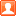# 平面设计师发表于 2015-10-7 21:17:31 | 显示全部楼层 |阅读模式

① 若该简历是广告或无意义简历，请向网站客服举报！
② 价格低廉、在更多信息里填写电话的要谨慎！
③ 若遇上疑似或确定为欺诈交易、网络诈骗等信息，请向本网站举报！

2=印前制版/排版/制作
2.1=制作/排版员
2.2=印前处理
2.3=拼版/CTP/菲林输出员
2.5=数码印刷/打样
2.6=电分/扫描
2.7=制图/修图
2.8=文案/校对
2.9=学徒
3 =生产/经营管理
3.1=生产厂长
3.2=车间主任
3.3=生产调度
3.4=业务经理
3.5=技术/质量主管
3.6=数码店长
3.7=销售/市场/业务员
3.8=前台//客服/文员/行政/人事/经理助理
4=印刷
4.1=印刷机长
4.2=印刷副手
4.4=印刷辅助
4.5=印刷学徒
5=装订
5.1=折页机工/胶订机工/三面切书机工
5.2=装订线/覆膜/模切
5.4=锁线/骑订工
5.6=精装师傅
5.8=装订学徒
6=包装加工
6.1=裱纸上光/过油
6.3=烫金
6.5=糊盒
6.7=模切/压痕
6.9=塑料包装
7=采购/物流/仓储
7.1=采购员
7.2=仓库管理员
7.3=送货员/外勤
7.4=保安/门卫
7.5=厨师/保洁
8=财务/审计/统计
8.1=会计
8.2=出纳
8.3=统计员
8.4=财务主管
8.5=审计

 平面设计师平面设计师平面设计师平面设计师平面设计师平面设计师平面设计师平面设计师平面设计师平面设计师平面设计师平面设计师平面设计师平面设计师平面设计师平面设计师平面设计师平面设计师平面设计师平面设计师平面设计师平面设计师平面设计师平面设计师平面设计师平面设计师平面设计师平面设计师平面设计师平面设计师平面设计师平面设计师平面设计师平面设计师平面设计师平面设计师平面设计师

### 本帖子中包含更多资源

x
 使用 高级模式（可批量传图、插入视频等） 回帖后跳转到最后一页
-->